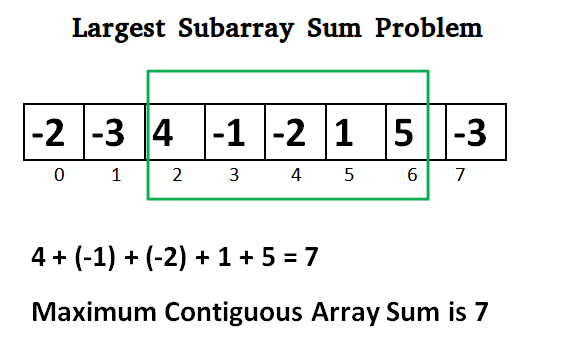# C / C++ Program for Largest Sum Contiguous Subarray

Write an efficient program to find the sum of contiguous subarray within a one-dimensional array of numbers which has the largest sum.## C++

 `// C++ program to print largest contiguous array sum ` `#include ` `#include ` `using` `namespace` `std; ` ` `  `int` `maxSubArraySum(``int` `a[], ``int` `size) ` `{ ` `    ``int` `max_so_far = INT_MIN, max_ending_here = 0; ` ` `  `    ``for` `(``int` `i = 0; i < size; i++) { ` `        ``max_ending_here = max_ending_here + a[i]; ` `        ``if` `(max_so_far < max_ending_here) ` `            ``max_so_far = max_ending_here; ` ` `  `        ``if` `(max_ending_here < 0) ` `            ``max_ending_here = 0; ` `    ``} ` `    ``return` `max_so_far; ` `} ` ` `  `/*Driver program to test maxSubArraySum*/` `int` `main() ` `{ ` `    ``int` `a[] = { -2, -3, 4, -1, -2, 1, 5, -3 }; ` `    ``int` `n = ``sizeof``(a) / ``sizeof``(a); ` `    ``int` `max_sum = maxSubArraySum(a, n); ` `    ``cout << ``"Maximum contiguous sum is "` `<< max_sum; ` `    ``return` `0; ` `} `

Output:

```Maximum contiguous sum is 7
```

Above program can be optimized further, if we compare max_so_far with max_ending_here only if max_ending_here is greater than 0.

## C++

 `int` `maxSubArraySum(``int` `a[], ``int` `size) ` `{ ` `    ``int` `max_so_far = 0, max_ending_here = 0; ` `    ``for` `(``int` `i = 0; i < size; i++) { ` `        ``max_ending_here = max_ending_here + a[i]; ` `        ``if` `(max_ending_here < 0) ` `            ``max_ending_here = 0; ` ` `  `        ``/* Do not compare for all elements. Compare only    ` `          ``when  max_ending_here > 0 */` `        ``else` `if` `(max_so_far < max_ending_here) ` `            ``max_so_far = max_ending_here; ` `    ``} ` `    ``return` `max_so_far; ` `} `

Please refer complete article on Largest Sum Contiguous Subarray for more details!

My Personal Notes arrow_drop_up
Article Tags :

Be the First to upvote.

Please write to us at contribute@geeksforgeeks.org to report any issue with the above content.